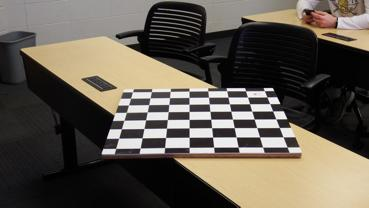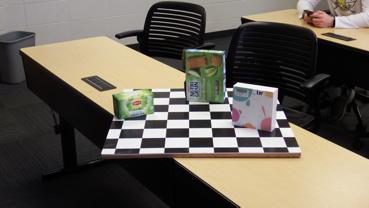# ECE 564 Computer Vision Assignment 5

Submit HTML documentation and MATLAB code on Isidore.

1. Calculate the focal length of the MATLAB camera from images from hall model4, which are stored in results.zip.
1. Plot the ratio of actual width to pixel width vs. distance to find the focal length. Use this data to determine the numerical value of focal length used in the MATLAB script.
2. Use the MATLAB calibrator to find the result. Warning, this will not work because the solution is indeterimate. Look at the uncertainty in focal lengths. Check the value of distances obtained from the extrinsics. You should find they differ by a single scaling factor, which should also correct the focal length.
3. Calibrate the MATLAB camera using the calibration toolbox with images from hall model4 (extended), which are stored in results2.zip. Note that the distortion parameters should be zero. Compare the focal length that obtained in the previous problem. Also compare the target locations (from extrinsics) to the MATLAB values.

2. Use the images taken in class 24-Feb-2020 to generate figures similar to those for Room KL351G. Compare explictly the results from extrinsics to our measurements. For example, where is the camera in the room? What are the dimensions of the room?

3. Using one of the table-top images (see sample below), get the extrinsics for the image. Then use the extrinsics and MATLAB camera functions to create a model corresponding as closely to the image as possible. The projection should be perspective, and the angle should match my camera.4. Using one of the table-top images, with your product, create a model that positions and orients your product in the same way. Results of the previous problem should be useful.5. Use `fitgeotrans` and `imwarp` to generate an ortho view of one of the checkerboard images. You may crop the results to show just the region of the target board. You can use `pat2s.jpg` to obtain reference points, or you can use the imagePoints/worldPoints.

6. Use the results of the previous problem to find the projective transform. Then read Hartley and Zisserman, Section 4.1, and write a MATLAB function for the direct linear transformation (DLT) algorithm. The result should match the matrix generated by `fitgeotrans` or `cp2tform` within a multiplicative factor.

#### Reference

Richard Hartley and Andrew Zisserman, Multiple View Geometry in Computer Vision, Cambridge, 2000. ISBN 0-521-54051-8.

Maintained by John Loomis, last updated 26 February 2019